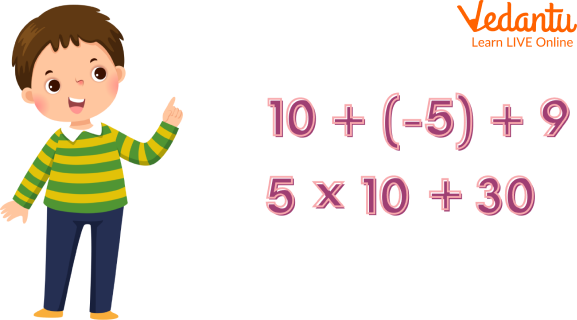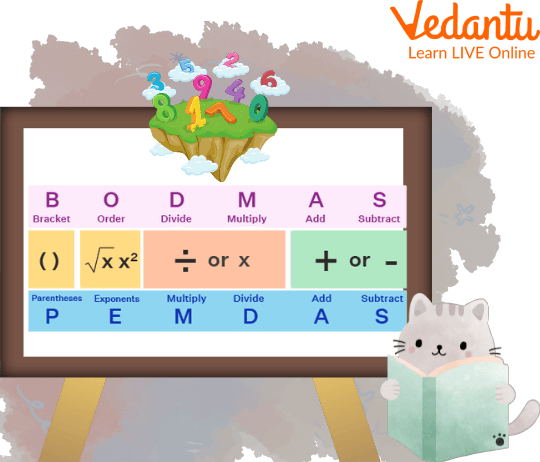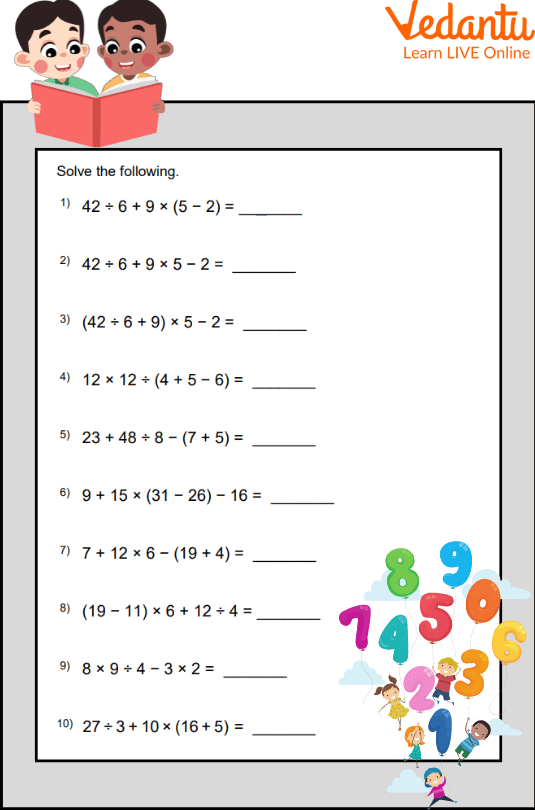Courses
Courses for Kids
Free study material
Free LIVE classes
More

# Operation In MathematicsLIVE
Join Vedantu’s FREE Mastercalss

## A Brief Explanation of Operation In Mathematics

Mixed operations are a set of operations in mathematics that can be defined as two or more operations, performed simultaneously on the same set of numbers. These are important to study in mathematics. Mixed operations are multiple operations involved like addition, subtraction, multiplication and division. Using PEMDAS or BODMAS rules, we demonstrate how to implement mixed operations. This article also helps you in attaining addition, subtraction, multiplication, and division worksheets pdf for free. So, let's begin.

## What are Mixed Operations?

Mixed operations are those operations in which more than one operation is done. Suppose there is an expression, 5 + 2 -1. Here we can see that two operations are used. One is addition and the other one is subtraction. So such expressions will come under the mixed operations.Mixed Operations

## Order of Operation

The below-given image shows the order in which the operations are applied to a problem, having more than one operation.Order of Operations

## Mixed Operations Rules

Following are the mixed operation rules:

Operation rule 1: First thing to do while operating the expression is to solve the numbers inside the parenthesis or bracket. We solve the parenthesis inside to out, grouping the operations. Observe the pattern of brackets present in the expression. There is an order to solve brackets, that is $[\{()\}]$. First, we solve the round bracket (), then the curly bracket {} then the box(square) bracket []. The order of operations to be followed inside the brackets.

Operation rule 2: After operating on parenthesis, we look for exponents. If present, solve them.

Operation rule 3: Now, we operate on the four basic operations. In this step, we look for numbers with multiplication and division operations. If present, solve them from left to right.

Operation rule 4: Last operations to be carried out are addition or subtraction and solving them from left to right.

Q 1. $4 \times(5+2)$

Ans: $4 \times(7)=28$ (Correct $(\checkmark)$.) This is a correct way to solve the parentheses) Let us look at another approach for the same expression. $4 \times(5+2)=20+2=22$ (Incorrect $(X)$.) This is an incorrect way to solve.

Q 2. $4 \times(-5)^2$

Ans: $4 \times(25)=100$ (Correct $(\checkmark)$.) This is a correct way to solve the exponents)

Another approach for the same expression may be as follows

$4 \times(-5)^2=-20^2=-400$ ((Incorrect $(X)$.) This is an incorrect way to solve the exponent

Q 3. $3+5 \times 2$

Ans: $3+5 \times 2=3+10=13$ (Correct $(\checkmark)$. Correct order.)

Another approach for the same expression may be as follows

$3+5 \times 2=8 \times 2=16$ (Incorrect $(X)$. Incorrect order.)

## Practice Problems

Here are some practice problems based on the addition and subtraction worksheets;

Q 1: Find the total of the difference of 230 and 101, with 452.

Ans. 581

Q 2. Find the sum of the difference of 435 and 281, with 412.

Ans. 566

Q 3. Subtract the sum of 79 and 53 from 123.

Ans. 9

Q 4. Multiply the quotient of 16 and 2 by 66.

Ans. 528

Q 5. Divide the multiplication of 6 and 3 by 9.

Ans. 2

## Mixed Operations Worksheets

This article provides you with the addition, subtraction, multiplication, and division worksheets pdf to practice more and more to gain proficiency.Mixed operations worksheets

## Summary

Mixed operation is a mathematical concept that is used in many different fields of mathematics. It is used to describe situations where an operation produces more than one result, and can be viewed as a combination of two or more different operations. This concept can be applied to many fields such as combinatorics, probability and statistics. In this article, we tried to discuss some of these mixed operations in mathematics terms, along with some solved mixed addition and subtraction word problems for grade 2. If you understand the concept, you may find it interesting to solve the given addition and subtraction worksheets, even if you don't like studying mathematics.

Last updated date: 27th Sep 2023
Total views: 78.6k
Views today: 1.78k

## FAQs on Operation In Mathematics

1. What are arithmetic operations?

Arithmetic operations are the basic operations which are used to perform simple calculations, such as addition, subtraction, multiplication or division. Arithmetic operations are represented using the symbols, +, -, ×, and ÷.

2. What are operations in mathematics?

Operations in mathematics are defined as the symbols used to combine two or more numbers. These operations are used to operate two numbers, known as operands, using one or two operations.

3. How can mixed operations in mathematics be helpful to students?

The mixed operations in mathematics can be helpful to students in many ways. For instance, it helps them understand the relationship between different types of numbers and their properties. It also helps them understand how to create new numbers from existing ones. The mixed operations help students learn how to think mathematically.